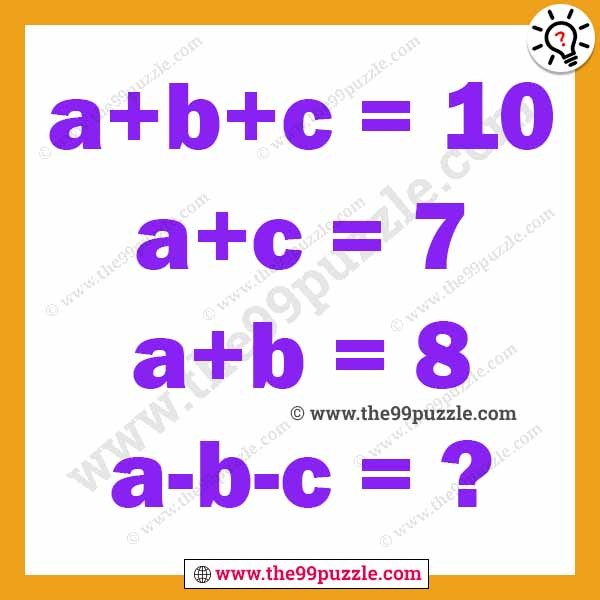Can you solve the adults logic brain teaser? This type of puzzle can challenge your logical mind. Observe the letter puzzles and solve it. If you are good puzzler you can quickly solve the math logic brain teaser for adults.

a+b+c=10

a+c=7

a+b=8

a-b-c=?a=5

b=3

c=2

a+b+c=5+3+2=10

a+c=5+2=7

a+b=5+3=8

a-b-c=5-3-2=0